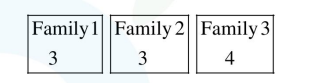# Two families with three members each and one family with four members are to be seated in a row. In how many ways can they be seated so that the same family members are not separated ?

Question:

Two families with three members each and one family with four members are to be seated in a row. In how many ways can they be seated so that the same family members are not separated ?

1. $2 ! 3 ! 4 !$

2. $(3 !)^{3} \cdot(4 !)$

3. $(3 !)^{2} \cdot(4 !)$

4. $3 !(4 !)^{3}$

Correct Option: , 2

Solution:

Total numbers in three familes $=3+3+4=10$ so total arrangement $=10$ !Favourable cases

$=$ $3 !$    $3 ! \times 3 ! \times 4 !$

$\therefore$ Probability of same family memebers are

together $=\frac{3 ! 3 ! 3 ! 4 !}{10 !}=\frac{1}{700}$

so option(2) is correct.